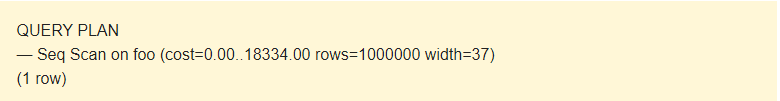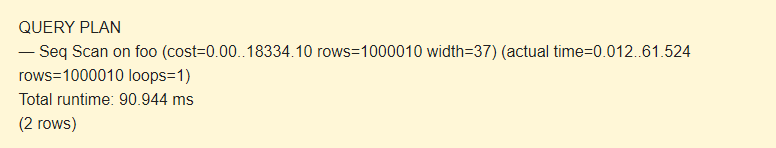09:19

# Query Optimization in PostgreSQL. EXPLAIN Basics – Part 1

Why does it take so much time to execute a query? Why are there no indexes? Chances are you’ve heard about EXPLAIN in PostgreSQL. However, there are still many people who have no idea how to use it. I hope this article will help users tackle with this great tool.

This article is the author revision of Understanding EXPLAIN by Guillaume Lelarge. Since I have missed out some information, I highly recommend you get acquainted with the original.

### The devil is not as black as he is painted

It is important to understand the logic of the PostgreSQL kernel to optimize queries. I’ll try to explain. It’s really not that complicated.

EXPLAIN displays the necessary information that explains what the kernel does for each specific query.

Let’s take a look at what the EXPLAIN command displays and understand what exactly happens inside PostgreSQL. You can apply this information to PostgreSQL 9.2 and higher versions.

• Learn how to read and understand the output of the EXPLAIN command
• Understand what happens in PostgreSQL when a query is executed

### First steps

We will be practicing on a test table with a million of rows.

```CREATE TABLE foo (c1 integer, c2 text);
INSERT INTO foo
SELECT i, md5(random()::text)
FROM generate_series(1, 1000000) AS i;```

`EXPLAIN SELECT * FROM foo;`It is possible to read data from a table in several ways. In our case, EXPLAIN notifies that a Seq Scan is used — a sequential, block-by-block, read Foo table data.

What is cost?

Well, it is not a time, but a concept designed to estimate the cost of an operation. The first value 0.00 is the costs for getting the first row. The second value 18334.00 is the costs for getting all the rows.

Rows are the approximate number of rows returned when a Seq Scan operation is performed. The scheduler returns this value. In my case, it matches the actual number of rows in the table.

Width is an average size of one row in bytes.

Let’s try to add 10 rows.

```INSERT INTO foo
SELECT i, md5(random()::text)
FROM generate_series(1, 10) AS i;
EXPLAIN SELECT * FROM foo;```The value of rows has not been changed. The statistics of the table is old. To update the statistics, call the ANALYZE command.

Now, rows display the correct number of rows.

### What happens when executing ANALYZE?

• Randomly, a number of rows are selected and read from the table.
• The statistics of values by each column is collected.

The number of rows ANALYZE reads depends on the default_statistics_target parameter.

### Actual data

Everything we saw above in the output of the EXPLAIN command is what the planner expects to get. Let’s try to compare them with the results on actual data. To do this, use EXPLAIN (ANALYZE).

`EXPLAIN (ANALYZE) SELECT * FROM foo;`Such a query will be performed indeed. So, if you execute EXPLAIN (ANALYZE) for the INSERT, DELETE, or UPDATE statements, your data will be changed. Be careful! In these cases, use the ROLLBACK command.

The command displays the following additional parameters:

• actual time is the actual time in milliseconds spent to get the first row and all rows, respectively.
• rows is the actual number of rows received with Seq Scan.
• loops is the number of times the Seq Scan operation had to be performed.
• Total runtime is the total time of query execution.